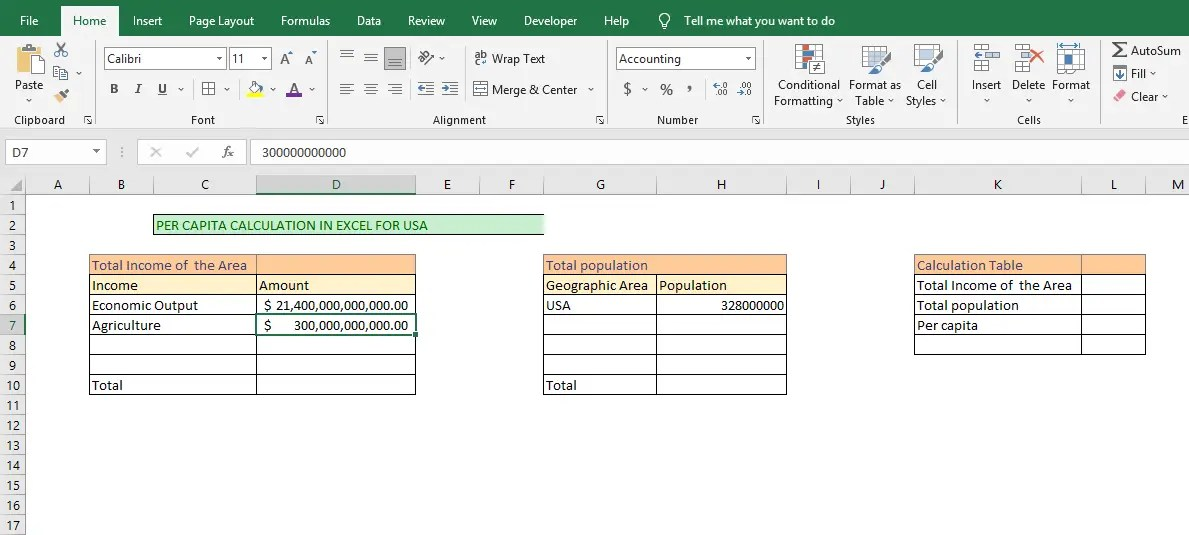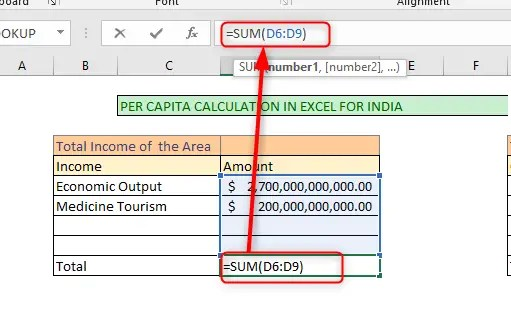# How to calculate per capita in Excel

Per capita is basically the amount of money each person earns in a specific nation or geographical region. This amount is used as a barometer in determining the average income that a person earns. It is able to evaluate a group of individuals’ standard of living in a geographic region, whether it is a country, city, sector, or area. Et.c. These calculations can be done in excel.

Here is the formula

Per Capital Income = Total Income of Area/Total population

The formula consists of these two parts the total population and the total income that all the individuals in these areas earn. It is calculated by the division of the area’s total income by the people that are living in this particular geographical area.

Say the total income earned by 10000 people in Minnesota is \$ 70000000. Then Per capita will be?

Per Capita= \$70000000/10000= \$7000

## Steps to calculate per Capita in Excel

To calculate per capita in Excel, you will need to understand how to arrange the questions in a systematic manner. See example

In 2019, India’s population was 1.36 trillion, with its general economic output being valued at \$2.7trillion and \$200billion for medical tourism. On the other hand, the US population was around 328 million, with an general economic output valued at \$21.4 trillion and \$300 billion for agriculture. Compare India and US per capita.

1. Classify to understand the total income area and the total population.

2. Launch excel and open the worksheet that you want to calculate per capita in excel

3. Create three tables in an open workbook (Total income of the area, total population, and Calculation table). See Screenshot example4. In the Total Income of the area and total population tables, insert all the items that belong to the table and their corresponding amount and population, respectively.

5. The table would look like this. For India and the US.6. Calculate the Total income of the area and the Total population using the SUM function. The total income of the area calculation will be= SUM (D6:D9).7. Calculate the Total population using the SUM function. The total population calculation will be =SUM (H6:H9).8. In the calculation table, insert the Total income of the area and the total population. For the Total income of the area cell L5, it will be =D\$10 and cell =H\$10 using conditional formatting.9. In the Calculation table, calculate the Per capita of the countries. Then Click cell L7. Insert the formula =L5/L610. There you go; the per capita for India is \$2.13 for India. Repeat the same for the US, and the US per capita is \$66,158.54. Comparing the two countries, the US had a higher income per capita as compared to India.

It is important to understand that per capita is an important barometer for the economy. With per capita

• It becomes easy to know the area’s wealth or scarcity. The indicator becomes important in arriving at an important socio-economic decision.
• It is also able to assess the affordability of the area which is the buying power of these individuals.
• It also ascertains the revenue of the region. Thus, helpful for organizations and business people to get an understanding of whether to open businesses or stores in the region.
• The government can also use the data to make socioeconomic decisions.